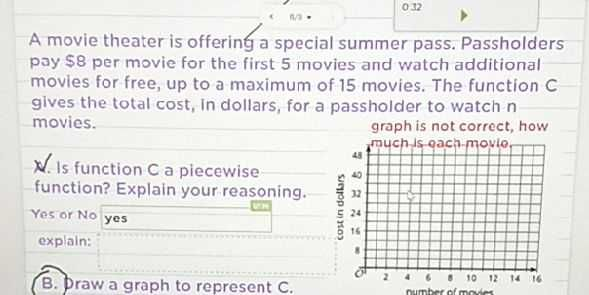### ¿Todavía tienes preguntas de matemáticas?

Pregunte a nuestros tutores expertos
Algebra
PreguntaA movie theater is offering a special summer pass. Passholders pay $$\ 8$$ per movie for the first $$5$$ movies and watch additional movies for free, up to a maximum of $$15$$ movies. The function C gives the total cost, in dollars, for a passholder to watch $$n$$ movies.

Q. Is function C a piecewise function? Explain your reasoning.

$$C(n)= \left\{\begin{matrix}8n,0\le n\le5\\40,5< n\le 15\end{matrix}\right.$$